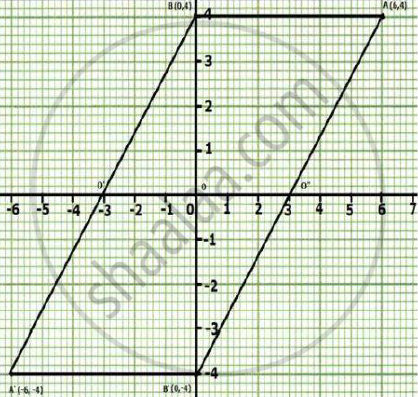# Using a graph paper, plot the point A (6, 4) and B (0, 4). (a) Reflect A and B in the origin to get the image A’ and B’. (b) Write the co-ordinates of A’ and B’ - Mathematics

Using a graph paper, plot the point A (6, 4) and B (0, 4).

(a) Reflect A and B in the origin to get the image A’ and B’.

(b) Write the co-ordinates of A’ and B’.

(c) Sate the geometrical name for the figure ABA’B’.

(d) Find its perimeter.

#### Solution

(a)(b) Co-ordinates of A'=(-6,-4)

Co-ordinates of B'=(0,-4)

(c) ABA'B' is parallelogram.

(d) In ABA'B',BB'=8 units, A'B'=6 units

therefore BA'=sqrt(6^2+8^2)=sqrt(36+64)=sqrt100=10 units

=>B'A=10  units

AB=A'B'=6units

∴ the perimeter of ABA'B'=AB+BA'+A'B'+B'A

=6+10+6+10

=32 units

Concept: Invariant Points.
Is there an error in this question or solution?

#### APPEARS IN

Selina Concise Maths Class 10 ICSE
Chapter 12 Reflection
Exercise 12 (B) | Q 15 | Page 171